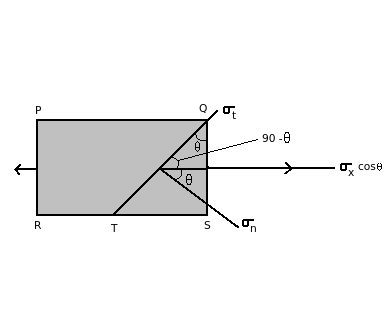# Strength of Materials - Mechanical Engineering (MCQ) questions and answers for Q. 11478

Q.  When a component is subjected to axial stress the normal stress σn is maximum, if cos θ is _______ . (σnxCos2θ)

1. maximum
2. minimum
3. always one
4. always zero

- Published on 21 Sep 15

a. 1 and 4
b. 1 and 3
c. 2 and 3
d. 2 and 4

#### Discussion

• Sravanthi   -Posted on 25 Nov 15- In the above diagram, on plane QS the stress acting is σx and the stress acting on plane QT is σx cos θ

- The normal stress acting is given σn = σx cos2 θ

- When cos θ is maximum, normal stress is maximum. Hence the maximum value of cos θ is always one.

- When cos θ = 1, θ = 0

## ➨ Post your comment / Share knowledgeEnter the code shown above:

(Note: If you cannot read the numbers in the above image, reload the page to generate a new one.)